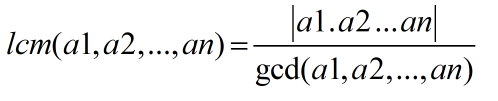Request a Tool

LCM Calculator

LCM Calculator is use to calculate least common multiple of input data set numbers.

LCM
0

Formula• lcm(a1,a2,...,an) = least common multiple of data set values
• gcd(a1,a2m...,an) = greatest common divisor of data set values
• a1.a2...an = Multiply of data set values

Defination / Uses

The "smallest non-zero common number" that is a multiple of both numbers is the "least common multiple of two numbers." The following are the several ways for determining the least common multiple of two or more numbers: Prime factorization is used.

The smallest positive integer that is divisible by each number in a set of integers (a, b, c...) is the least common multiple, also known as the lowest common multiple or smallest common multiple. We just have two numbers, a and b, in the simplest example, and we may use the notation LCM (a, b). The LCM is also known as the "lowest common denominator," which must be discovered before adding, subtracting, or comparing fractions (see our LCD calculator). Using our LCM calculator on your mobile or desktop computer, you can quickly calculate the least common multiple.